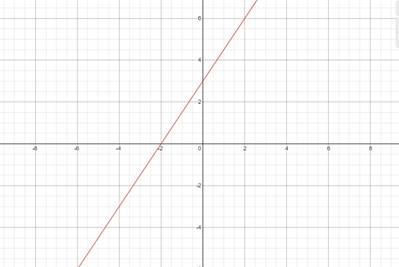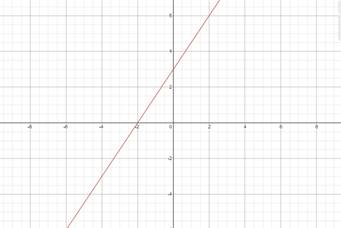# Slope and y-intercept of given equation, plot its graph### Precalculus: Mathematics for Calcu...

6th Edition
Stewart + 5 others
Publisher: Cengage Learning
ISBN: 9780840068071### Precalculus: Mathematics for Calcu...

6th Edition
Stewart + 5 others
Publisher: Cengage Learning
ISBN: 9780840068071

#### Solutions

Chapter 1.10, Problem 49E
To determine

## Slope and y-intercept of given equation, plot its graph

Expert Solution

Slope=32yintercept=3### Explanation of Solution

Given information:

12x13y+1=0

Formula used:

Slope-intercept form of line: y=mx+c , where m is the slope and c is the y-intercept cut by the line.

12x13y+1=0

Converting the line into slope intercept form

x2y3+1=0y3=x2+1y=3x2+3

m=32c=3

Plotting the Graph

Finding two points on line

x=662y3+1=03+1=y3y=12

(6,12)

y=6x263+1=0x2=21x=2

(2,6)### Have a homework question?

Subscribe to bartleby learn! Ask subject matter experts 30 homework questions each month. Plus, you’ll have access to millions of step-by-step textbook answers!